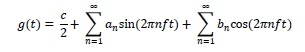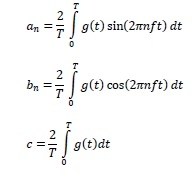# What is a Fourier Analysis?

Fourier analysis is a method of representing general functions by approximate sum of simple trigonometric functions. The method is named after mathematician Jean Baptiste Joseph Fourier who formulated and proved the Fourier series. Fourier analysis is used in electronics, communications and acoustics.

The Fourier series decomposes a periodic function as a sum of sine and cosine components as expressed below:where, g(t) is the periodic function

T is the time period

f is the fundamental frequency expressed as 1/T

an is the sine amplitude of the nth harmonic

bn is the cosine amplitude of the nth harmonic

c is a constant

The values of an, bn and c are computed by the following expressions: